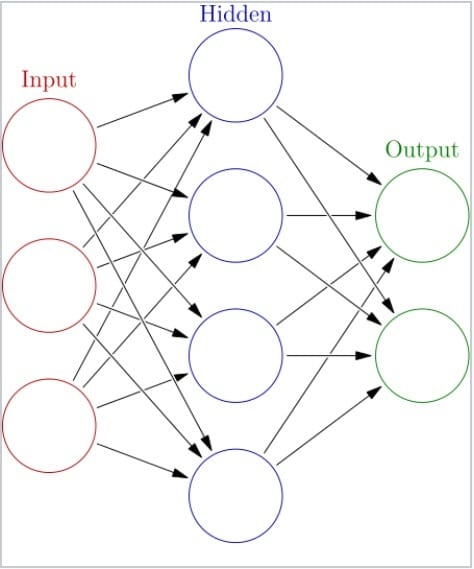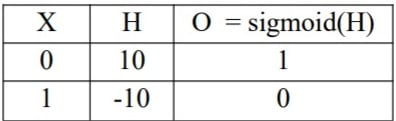# Neural NetworkPosted by

(If you have not gone through my first article on , please go through it. It would be really helpful to understand this one.)

Hello folks, Greetings of the day!!!
Good to see you again 🙂
In the last article, we had a small trip to the magical world of Machine Learning. (It was very interesting. Isn’t it?)   Let’s have another small trip to that magical world of Machine Learning again. (Hurreeyy!!!)

Earlier we discussed about what Machine Learning actually means, how it differs from the traditional programming and two basic types of Machine Learning i.e. Supervised and Unsupervised. In this article, we are going to discuss about very fundamental and heart of the machine learning – Neural Network. (One more interesting and magical word. Right?)   So let’s start…

### What is Neural Network?

Neural Network is a computing system inspired by biological neural network that constitute animal brains. Such systems learn to perform tasks by considering examples, generally without programmed with task specific rules.

(Definition Source : https://en.wikipedia.org/wiki/Artificial_neural_network)

Let’s take an example of human brain. How child learn to walk? (Here walking is task T) He see and observe others walking and then he tries to walk himself. He may stumble in starting phase but eventually he will learn to walk perfectly (It is experience E) and after having numerous experiences, He will be walking perfectly (It is performance P). The same is with Neural Networks of machines. It takes the task, learns from experiences and finally, performs the task accurately.

That was all about theoretical terms. Now let’s understand what neural networks means in mathematical terms i.e. Machine Learning

### Basic Model of Neural NetworkImage Source : WiKi

Here is the very basic model of Neural Network. There are mainly three layers i.e. Input layer, Hidden Layer (can be more than one) and Output layer. Suppose inputs are X1, X2, X3, hidden layer values H1, H2 , H3 , H4 and outputs O1 and O2.

There is mathematical relation between next layer and previous  layer.

H1 = f(X1, X2, X3), same for H2 , H3 and H4 .

O1 = g1(H1, H2 , H3 , H4 ) and O2 = g2(H1, H2 , H3 , H4 )

Now let’s take some simple examples of Neural Network by implementing logical gate. Yes!!! We can implement logical gates by Neural Network.

### OR Gate

Before we start with OR gate, let me brief you about the sigmoid function. The equation and the graph of the sigmoid function are given below:So here is the Neural Network to implement the logical OR Gate.where H = -10 + 20X1 + 20X2, sigmoid(-10)=0, sigmoid(10)=1, sigmoid(30)=1. (You may refer the graph of sigmoid  functions for these values) .From above truth table, one can easily understand how Neural Network of logical OR gate works.

You might have few questions in your mind. Why sigmoid function only? Why not any other function?

So the answer to your questions is the range of the sigmoid function is between 0 to 1.(As we can see from the above graph.)  As here we wanted to implement logical OR gate, the range of output is either 0 or 1.So we have used the sigmoid function. The functions may vary with the application and functioning of the Neural Network.

Here we have given +1 in input which is known as bias value. It may be or may not be present in Neural Network. (Depends on the application)

### NOT Gate

Same as OR gate, we can also implement NOT gate.Here  H = 10 -20X### NOR Gate

Now implementing logical NOR gate by combining the Neural Network of OR gate and NOT gate.Consider here H1 = sigmoid (-10+20X1+20X2) and H2 = (10-20H1).This is how we can merge two or more Neural Networks to implement another Neural Network.

So these were the basic examples of the Neural Network. More complex and intelligent Neural Network can be implemented by having multiple hidden layers and different relations between the values of two layers.

That’s it!!! See, you have learned so many basic concepts of Machine Learning so far.

Keep learning, wish you good luck! 🙂

###Monil  is an Electronics & Communications Engineering  final year student at Institute of Technology, Nirma University, Ahmedabad . In initial years, He  did numerous projects on Arduino and Raspberry Pi board like Surveillance on vehicle, Automatic Obstacle Detection on Vehicle, Automatic Traffic Routing, Automatic Railway Gate Control and few more. As of now, He is  working on project Self-Driving Car.  His fields of interests are Machine Learning, Computer Vision and Embedded Systems.  His field of interest are Not only technical aspects, But he has been also a part of Core Committee and managed the National Tech Fest , Cultural fest etc in the college. He likes to help others in learning technical aspects or in case of any problems.

You may  find interesting topics at